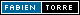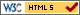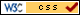•   ▽       fast access       ▽

# JStumps: boosting of stumps in Java

JStumps implements in Java the boosting of stumps. Many options are available and described below.

## Examples

### JStumps program

#### Learning in verbose mode

```# java JStumps --file=datasets/breast-cancer/CrossValidation/shot_1 --boost=20
. parameters used:
help        = false
verb        = true
save        = false
test        = false
isoclass    = false
file        = /home/torre/datasets/breast-cancer/shot_1
insist      = 10
onthefly    = false
boost       = 20
showst      = false
. 20 steps of boosting now
. 18 stumps found
. error rate on data file: 3,97 %
```

#### Learn and test, not verbose

```# java JStumps --file=datasets/breast-cancer/CrossValidation/shot_1 --boost=20 --noverb --test
. error rate on test file: 4,35 %
```

#### Learn and save classifier

```# java JStumps --file=datasets/breast-cancer/CrossValidation/shot_1 --boost=20 --noverb --save
```

### JSConsult program

#### Consult classifier

```# java JSConsult --classifier=datasets/breast-cancer/CrossValidation/shot_1.JStumps --noverb --showst
if UniformityCellSize<=3.0 then class 2 else class 4 [weight=0.17779300366525003]
if ClumpThickness<=6.0 then class 2 else class 4 [weight=0.13548074159737228]
if BareNuclei<=1.0 then class 2 else class 4 [weight=0.10766897146164137]
if UniformityCellSize<=3.0 then class 4 else class 2 [weight=0.09351568652457329]
if BlandChromatin<=2.0 then class 2 else class 4 [weight=0.06902295191295167]
if NormalNucleoli<=3.0 then class 2 else class 4 [weight=0.04925353047208494]
if BlandChromatin<=4.0 then class 2 else class 4 [weight=0.047019527580647674]
if MarginalAdhesion<=1.0 then class 2 else class 4 [weight=0.0361683906745061]
if Mitoses<=1.0 then class 2 else class 4 [weight=0.034611098925205766]
if UniformityCellShape<=2.0 then class 2 else class 4 [weight=0.03309250317288488]
if ClumpThickness<=2.0 then class 2 else class 4 [weight=0.03203251573592544]
if ClumpThickness<=4.0 then class 2 else class 4 [weight=0.03196929631350762]
if NormalNucleoli<=1.0 then class 4 else class 2 [weight=0.029934655990969988]
if ClumpThickness<=5.0 then class 4 else class 2 [weight=0.028165092442751286]
if BareNuclei<=3.0 then class 2 else class 4 [weight=0.02730910998261713]
if SingleEpithelialCellSize<=1.0 then class 4 else class 2 [weight=0.023181867572012904]
if SingleEpithelialCellSize<=2.0 then class 2 else class 4 [weight=0.02212647398009039]
if MarginalAdhesion<=9.0 then class 2 else class 4 [weight=0.021654581995007294]
```

#### Classify test examples

```# java JSConsult --classifier=datasets/breast-cancer/CrossValidation/shot_1.JStumps
--file=datasets/breast-cancer/CrossValidation/shot_1 --noverb --predictions
test1,4,0.41566580533981323,4
test2,4,0.5130572319030762,4
test3,4,0.09624868631362915,4
test4,4,0.3285263776779175,4
test5,2,0.24965348839759827,4
test6,4,0.3390907943248749,4
test7,4,0.1266273856163025,4
test8,4,0.12250885367393494,4
test9,4,0.607096254825592,4
test10,4,0.44383499026298523,4
test11,4,0.5130572319030762,4
test12,4,0.3924649953842163,4
test13,4,0.6504054665565491,4
test14,4,0.5563663840293884,4
test15,4,0.2863062620162964,4
.............................
```

#### Output confusion matrix

```# java JSConsult --classifier=datasets/breast-cancer/CrossValidation/shot_1.JStumps
--file=datasets/breast-cancer/CrossValidation/shot_1 --noverb --confusion
;2,2,43;2,4,1;4,2,2;4,4,23
```

• use java JStumps --help
• use java JSConsult --help
• works for learning problems with two classes to predict only
• missing values are authorized in both training set and test set
• JStumps uses C4.5 formats (.names, .data and .test)

## Bibliography

Definition of stumps (also called AttrTest, section 3.1):

Yoav Freund and Robert E. Schapire
Experiments with a New Boosting Algorithm
1996
CiteSeer page ]

Boosting algorithm used in this implementation (Adaboost, figure 1):

Yoav Freund and Robert E. Schapire
A short introduction to boosting
IJCAI 1999
CiteSeer page ]Accueil > Research > Softs > JStumps
(last update )
Fabien Torre's site, university of Lille

#### Description

Survoler un lien de navigation pour lire sa description ici...